Precipitation ReactionsPage 1

WATCH ALL SLIDES

Slide 1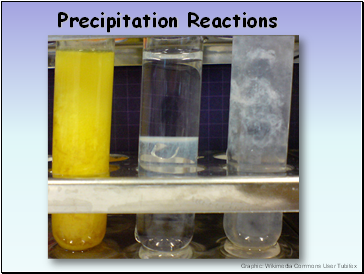Precipitation Reactions

Graphic: Wikimedia Commons User Tubifex

Slide 2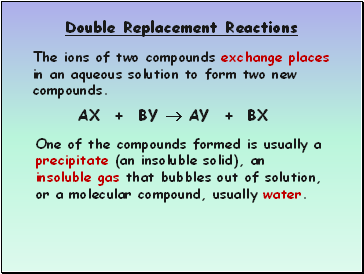Double Replacement Reactions

The ions of two compounds exchange places in an aqueous solution to form two new compounds.

AX + BY  AY + BX

One of the compounds formed is usually a

precipitate (an insoluble solid), an insoluble gas that bubbles out of solution, or a molecular compound, usually water.

Slide 3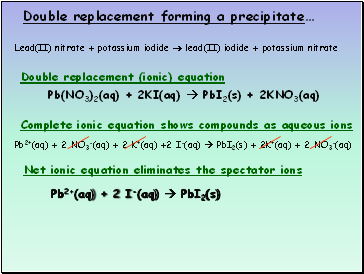Double replacement forming a precipitate…

Pb(NO3)2(aq) + 2KI(aq)  PbI2(s) + 2KNO3(aq)

Pb2+(aq) + 2 NO3-(aq) + 2 K+(aq) +2 I-(aq)  PbI2(s) + 2K+(aq) + 2 NO3-(aq)

Pb2+(aq) + 2 I-(aq)  PbI2(s)

Double replacement (ionic) equation

Complete ionic equation shows compounds as aqueous ions

Net ionic equation eliminates the spectator ions

Lead(II) nitrate + potassium iodide  lead(II) iodide + potassium nitrate

Slide 4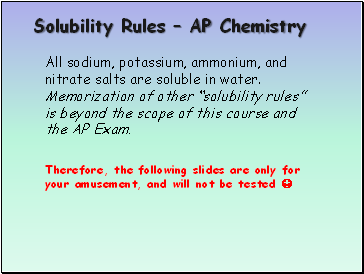Solubility Rules – AP Chemistry

All sodium, potassium, ammonium, and nitrate salts are soluble in water. Memorization of other “solubility rules” is beyond the scope of this course and the AP Exam.

Therefore, the following slides are only for your amusement, and will not be tested 

Slide 5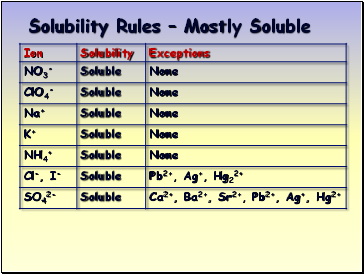Solubility Rules – Mostly Soluble

Slide 6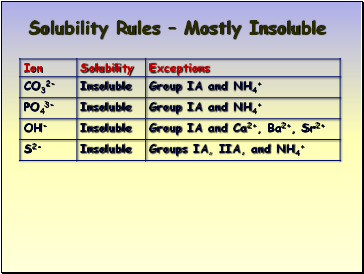Solubility Rules – Mostly Insoluble

Slide 7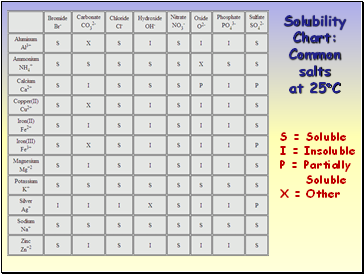Solubility Chart: Common salts at 25C

S = Soluble

I = Insoluble

P = Partially

Soluble

X = Other